# 209 Results

View
Selected filters:
• MI.Math.Practice.MP.4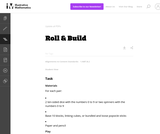Unrestricted Use
CC BY
Rating

This is a task from the Illustrative Mathematics website that is one part of a complete illustration of the standard to which it is aligned. Each task has at least one solution and some commentary that addresses important asects of the task and its potential use. Here are the first few lines of the commentary for this task: Materials For each pair: * 2 ten-sided dice with the numbers 0 to 9 or two spinners with the numbers 0 to 9 * Base-10 blocks, linking cubes, or bundled...

Subject:
Mathematics
Material Type:
Activity/Lab
Provider:
Illustrative Mathematics
Provider Set:
Illustrative Mathematics
Author:
Illustrative Mathematics
05/24/2013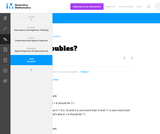Unrestricted Use
CC BY
Rating

This is a task from the Illustrative Mathematics website that is one part of a complete illustration of the standard to which it is aligned. Each task has at least one solution and some commentary that addresses important aspects of the task and its potential use.

Subject:
Algebra
Material Type:
Activity/Lab
Provider:
Illustrative Mathematics
Provider Set:
Illustrative Mathematics
Author:
Illustrative Mathematics
02/16/2018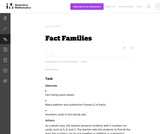Unrestricted Use
CC BY
Rating

This is a task from the Illustrative Mathematics website that is one part of a complete illustration of the standard to which it is aligned. Each task has at least one solution and some commentary that addresses important asects of the task and its potential use. Here are the first few lines of the commentary for this task: Materials * Fact Family work sheets * Blank addition and subtraction frames (2 of each) * Numbers cards in fact-family sets Actions As a whole class, t...

Subject:
Mathematics
Material Type:
Activity/Lab
Provider:
Illustrative Mathematics
Provider Set:
Illustrative Mathematics
Author:
Illustrative Mathematics
09/06/2013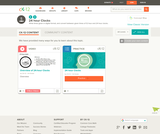Conditional Remix & Share Permitted
CC BY-NC-SA
Rating

This short video and interactive assessment activity is designed to give fifth graders an overview of 24-hour clocks.

Subject:
Mathematics
Numbers and Operations
Material Type:
Assessment
Interactive
Lecture
Provider:
CK-12 Foundation
Provider Set:
CK-12 Elementary Math
04/03/2018Unrestricted Use
CC BY
Rating

This is a task from the Illustrative Mathematics website that is one part of a complete illustration of the standard to which it is aligned. Each task has at least one solution and some commentary that addresses important asects of the task and its potential use. Here are the first few lines of the commentary for this task: Materials * Alexander, Who Used to be Rich Last Sunday by Judith Viorst * Plastic coins * Labels for items Alexander spent his money on (attached) * Pa...

Subject:
Mathematics
Material Type:
Activity/Lab
Provider:
Illustrative Mathematics
Provider Set:
Illustrative Mathematics
Author:
Illustrative Mathematics
06/23/2013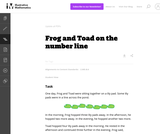Unrestricted Use
CC BY
Rating

This is a task from the Illustrative Mathematics website that is one part of a complete illustration of the standard to which it is aligned. Each task has at least one solution and some commentary that addresses important asects of the task and its potential use. Here are the first few lines of the commentary for this task: One day, Frog and Toad were sitting together on a lily pad. Some lily pads were in a line across the pond. In the morning, Frog hopped three lily pads ...

Subject:
Mathematics
Material Type:
Activity/Lab
Provider:
Illustrative Mathematics
Provider Set:
Illustrative Mathematics
Author:
Illustrative Mathematics
03/16/2013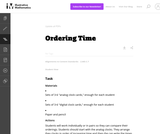Unrestricted Use
CC BY
Rating

This is a task from the Illustrative Mathematics website that is one part of a complete illustration of the standard to which it is aligned. Each task has at least one solution and some commentary that addresses important asects of the task and its potential use. Here are the first few lines of the commentary for this task: Materials * Sets of 3-6 "analog clock cards," enough for each student * Sets of 3-6 "digital clock cards," enough for each student * Paper and pencil A...

Subject:
Mathematics
Material Type:
Activity/Lab
Provider:
Illustrative Mathematics
Provider Set:
Illustrative Mathematics
Author:
Illustrative Mathematics
05/22/2013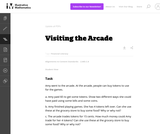Unrestricted Use
CC BY
Rating

This is a task from the Illustrative Mathematics website that is one part of a complete illustration of the standard to which it is aligned. Each task has at least one solution and some commentary that addresses important asects of the task and its potential use. Here are the first few lines of the commentary for this task: Amy went to the arcade. At the arcade, people can buy tokens to use for the games. Amy paid \5 to get some tokens. Show two different ways she could h... Subject: Mathematics Material Type: Activity/Lab Provider: Illustrative Mathematics Provider Set: Illustrative Mathematics Author: Illustrative Mathematics Date Added: 03/24/2013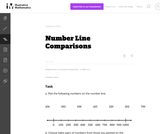Unrestricted Use CC BY Rating This is a task from the Illustrative Mathematics website that is one part of a complete illustration of the standard to which it is aligned. Each task has at least one solution and some commentary that addresses important asects of the task and its potential use. Here are the first few lines of the commentary for this task: Plot the following numbers on the number line. 456 \ \ 983\ \ 938 \ \ 425 \ \ 220 \ \ 202\ \ 799 Choose eight pairs of numbers from those you plotted o... Subject: Mathematics Material Type: Activity/Lab Provider: Illustrative Mathematics Provider Set: Illustrative Mathematics Author: Illustrative Mathematics Date Added: 04/04/2014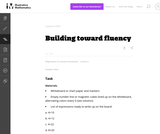Unrestricted Use CC BY Rating This is a task from the Illustrative Mathematics website that is one part of a complete illustration of the standard to which it is aligned. Each task has at least one solution and some commentary that addresses important asects of the task and its potential use. Here are the first few lines of the commentary for this task: Materials * Whiteboard or chart paper and markers * Empty number line or magnetic cubes lined up on the whiteboard, alternating colors every 5 (see sol... Subject: Mathematics Material Type: Activity/Lab Provider: Illustrative Mathematics Provider Set: Illustrative Mathematics Author: Illustrative Mathematics Date Added: 06/23/2013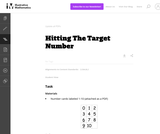Unrestricted Use CC BY Rating This is a task from the Illustrative Mathematics website that is one part of a complete illustration of the standard to which it is aligned. Each task has at least one solution and some commentary that addresses important asects of the task and its potential use. Here are the first few lines of the commentary for this task: Materials * Number cards labeled 1-10 (attached as a PDF) Actions * Begin by playing the game as a whole class to demonstrate the rules and for student... Subject: Mathematics Material Type: Activity/Lab Provider: Illustrative Mathematics Provider Set: Illustrative Mathematics Author: Illustrative Mathematics Date Added: 06/23/2013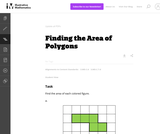Unrestricted Use CC BY Rating This is a task from the Illustrative Mathematics website that is one part of a complete illustration of the standard to which it is aligned. Each task has at least one solution and some commentary that addresses important asects of the task and its potential use. Here are the first few lines of the commentary for this task: Find the area of each colored figure. Each grid square is 1 inch long.... Subject: Mathematics Material Type: Activity/Lab Provider: Illustrative Mathematics Provider Set: Illustrative Mathematics Author: Illustrative Mathematics Date Added: 05/27/2013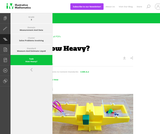Unrestricted Use CC BY Rating This is a task from the Illustrative Mathematics website that is one part of a complete illustration of the standard to which it is aligned. Each task has at least one solution and some commentary that addresses important aspects of the task and its potential use. Subject: Measurement and Data Material Type: Activity/Lab Provider: Illustrative Mathematics Provider Set: Illustrative Mathematics Author: Illustrative Mathematics Date Added: 02/16/2018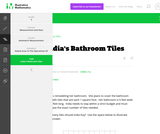Unrestricted Use CC BY Rating This is a task from the Illustrative Mathematics website that is one part of a complete illustration of the standard to which it is aligned. Each task has at least one solution and some commentary that addresses important aspects of the task and its potential use. Subject: Measurement and Data Material Type: Activity/Lab Provider: Illustrative Mathematics Provider Set: Illustrative Mathematics Author: Illustrative Mathematics Date Added: 02/16/2018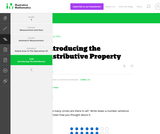Unrestricted Use CC BY Rating This is a task from the Illustrative Mathematics website that is one part of a complete illustration of the standard to which it is aligned. Each task has at least one solution and some commentary that addresses important aspects of the task and its potential use. Subject: Measurement and Data Material Type: Activity/Lab Provider: Illustrative Mathematics Provider Set: Illustrative Mathematics Author: Illustrative Mathematics Date Added: 02/16/2018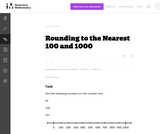Unrestricted Use CC BY Rating This is a task from the Illustrative Mathematics website that is one part of a complete illustration of the standard to which it is aligned. Each task has at least one solution and some commentary that addresses important asects of the task and its potential use. Here are the first few lines of the commentary for this task: Plot the following numbers on the number line: 80 328 791 1. Round each number to the nearest 100. How can you see this on the number line? 2. Round ea... Subject: Mathematics Material Type: Activity/Lab Provider: Illustrative Mathematics Provider Set: Illustrative Mathematics Author: Illustrative Mathematics Date Added: 02/18/2014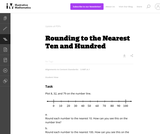Unrestricted Use CC BY Rating This is a task from the Illustrative Mathematics website that is one part of a complete illustration of the standard to which it is aligned. Each task has at least one solution and some commentary that addresses important asects of the task and its potential use. Here are the first few lines of the commentary for this task: Plot 8, 32, and 79 on the number line. 1. Round each number to the nearest 10. How can you see this on the number line? 2. Round each number to the nea... Subject: Mathematics Material Type: Activity/Lab Provider: Illustrative Mathematics Provider Set: Illustrative Mathematics Author: Illustrative Mathematics Date Added: 02/18/2014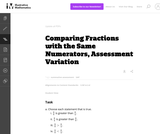Unrestricted Use CC BY Rating This is a task from the Illustrative Mathematics website that is one part of a complete illustration of the standard to which it is aligned. Each task has at least one solution and some commentary that addresses important asects of the task and its potential use. Here are the first few lines of the commentary for this task: Choose each statement that is true.\frac98$is greater than$\frac{9}{4}$.$\frac{9}{4}$is greater than$\frac98$.$\frac98 \gt \frac{9}{4}$.$\frac...

Subject:
Mathematics
Material Type:
Activity/Lab
Provider:
Illustrative Mathematics
Provider Set:
Illustrative Mathematics
Author:
Illustrative Mathematics
03/18/2013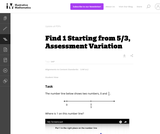Unrestricted Use
CC BY
Rating

This is a task from the Illustrative Mathematics website that is one part of a complete illustration of the standard to which it is aligned. Each task has at least one solution and some commentary that addresses important asects of the task and its potential use. Here are the first few lines of the commentary for this task: The number line below shows two numbers, 0 and $\frac53$. Where is 1 on this number line? http://www.youtube.com/watch?v=wAXRjMUgiu4...

Subject:
Mathematics
Material Type:
Activity/Lab
Provider:
Illustrative Mathematics
Provider Set:
Illustrative Mathematics
Author:
Illustrative Mathematics
03/18/2013Unrestricted Use
CC BY
Rating

This is a task from the Illustrative Mathematics website that is one part of a complete illustration of the standard to which it is aligned. Each task has at least one solution and some commentary that addresses important asects of the task and its potential use. Here are the first few lines of the commentary for this task: Alec and Felix are brothers who go to different schools. The school day is just as long at Felix' school as at Alec's school. At Felix' school, there a...

Subject:
Mathematics
Material Type:
Activity/Lab
Provider:
Illustrative Mathematics
Provider Set:
Illustrative Mathematics
Author:
Illustrative Mathematics